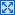# class Range < Object

A `Range` represents an interval—a set of values with a start and an end. Ranges may be constructed using the s`..`e and s`...`e literals, or with `Range.new`. Ranges constructed using `..` run from the start to the end inclusively. Those created using `...` exclude the end value. When used as an iterator, ranges return each value in the sequence.

```(-1..-5).to_a → [] (-5..-1).to_a → [-5, -4, -3, -2, -1] ('a'..'e').to_a → ["a", "b", "c", "d", "e"] ('a'...'e').to_a → ["a", "b", "c", "d"]```

Ranges can be constructed using objects of any type, as long as the objects can be compared using their `<=>` operator and they support the `succ` method to return the next object in sequence.

```class Xs # represent a string of 'x's include Comparable attr :length def initialize(n) @length = n end def succ Xs.new(@length + 1) end def <=>(other) raise TypeError unless other.kind_of? Xs @length <=> other.length end def inspect 'x' * @length end end r = Xs.new(3)..Xs.new(6) → xxx..xxxxxx r.to_a → [xxx, xxxx, xxxxx, xxxxxx] r.member?(Xs.new(5)) → true```

## mixins

`Enumerable`
`collect`, `detect`, `each_with_index`, `entries`, `find`, `find_all`, `grep`, `include?`, `map`, `max`, `member?`, `min`, `reject`, `select`, `sort`, `to_a`

## class methods

new
Range.new( start, end, exclusive`=false` ) → aRange
Constructs a range using the given start and end. If the third parameter is omitted or is `false`, the range will include the end object; otherwise, it will be excluded.

## instance methods

===
rng === anObject`true` or `false`
Returns `true` if anObject is an element of rng, `false` otherwise. Conveniently, `===` is the comparison operator used by `case` statements.
```case 79 when 1..50 then print "low\n" when 51..75 then print "medium\n" when 76..100 then print "high\n" end```

produces:

`high`
begin
rng.begin → anObject
Returns the first object of rng.
each
rng.each {| i | block } → rng
Iterates over the elements rng, passing each in turn to the block.
```(10..15).each do |n| print n, ' ' end```

produces:

`10 11 12 13 14 15`
end
rng.end → anObject
Returns the object that defines the end of rng. See also `Range#length`.
```(1..10).end → 10 (1...10).end → 10```
exclude_end?
rng.exclude_end? → `true` or `false`
Returns `true` if rng excludes its end value.
first
rng.first → anObject
Returns the first object in rng.
last
rng.last → anObject
Synonym for `Range#end`.
length
rng.length → anInteger
Returns the number of objects in rng.
```(1..10).length → 10 (1...10).length → 9```
size
rng.size → anInteger
Synonym for `Range#length`.